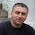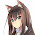## Saturday, February 11, 2012

### Haskell for Java Programmers - Serialization

Serialization is damn simple in Haskell. Just import Data.Binary (from the binary package) and use encodeFile/decodeFile on any object that implements the Binary interface:
```encodeStuff = do
encodeFile "Int.dat" (4 :: Int)
encodeFile "IntList.dat" ([1..3] :: [Int])
encodeFile "Boolean.dat" (True :: Bool)

decodeStuff = do
int     <- decodeFile "Int.dat"     :: IO Int
intList <- decodeFile "IntList.dat" :: IO [Int]
bool    <- decodeFile "Boolean.dat" :: IO Bool
return (int, intList, bool)
```
```> encodeStuff
> decodeStuff
(4,[1,2,3],True)
```
You usually don't need the type annotations in a non-trivial program as the compiler can infer their types for you.

Haskell's name for an interface is a "class" (and I know that's confusing for Java programmers). An "instance of a class" means implementing an interface in Haskell. Let's look up the documentation for the Binary "class" (i.e. interface) to see what methods it requires:
```class Binary where
put :: t -> Put
get :: Get t```
Anything can implement the Binary interface so long as it provides a serialization function (i.e. put) and a deserialization function (i.e. get). All you need to know about the Put and Get types is that they are "monads", which means that we can use Haskell do-notation to chain together larger put implementations from smaller ones. For example, we can implement the Binary interface for a generic tree structure:
```data Tree a = Leaf | Node a (Tree a) (Tree a) deriving (Show)

instance (Binary a) => Binary (Tree a) where
put x = case x of
Leaf -> put (0 :: Word8)
Node x l r -> do
put (1 :: Word8)
put x
put l
put r
get = do
t <- get :: Get Word8
case t of
0 -> return Leaf
1 -> do
x <- get
l <- get
r <- get
return (Node x l r)

> encodeFile "Tree.dat" (Node 1 Leaf Leaf :: Tree Int)
> decodeFile "Tree.dat" :: IO (Tree Int)
Node 1 Leaf Leaf
```
The first line of our class instance says:
`instance (Binary a) => Binary (Tree a) where`
...which means "If we can serialize an object of type a, we can serialize an object of type Tree a. This would be like Java's:
`public class Tree <A extends Binary> implements Binary`
put is an overloaded function that encodes anything that implements Binary. In our put definition, we use three different overloaded instance of put when encoding the Node constructor:
```put (1 :: Word8) -- put overloaded for Word8
put x            -- put overloaded for 'a'
put l            -- put overloaded for 'Tree a'
put r            -- put overloaded for 'Tree a'
```
Note that the last two calls to put are recursive. We define the put function for Tree a in terms of itself, structurally recursing over the tree. In the above example, we store the tree in preorder.

The compiler automatically inferred which overloaded version of put to use for the last three calls. The compiler infers the type of x, l, and r since they originated from the Node constructor:
`Node x l r -> do`
... so it knows that x must have type a, and l and r must have type Tree a. The first call to put explicitly gives a type signature for the 1 because numeric literals in Haskell are polymorphic, so you must explicitly state their type before passing them to overloaded functions.

Our get function also calls three different overloaded versions of get:
```t <- get :: Get Word 8 -- get overloaded for Word8
...
x <- get               -- get overloaded for 'a'
l <- get               -- get overloaded for 'Tree a'
r <- get               -- get overloaded for 'Tree a'
```
Again, the compiler infers the type of x, l, and r because it knows they will be used later on to assemble a Node:
`return (Node x l r)`
Actually, we can do much better than that. If you learn about the Applicative "class" (i.e. interface) and you implement it for Tree, you can dramatically simplify the definition of get by completely eliminating the intermediate variables x, l, and r:
```...
get = do
t <- get :: Get Word8
case t of
0 -> return Leaf
1 -> Node <\$> get <*> get <*> get
```
In other words, we just get the values directly into the Node constructor. Magic! Actually, it's not magic and Haskell doesn't use any hacks or language features or side-effects to make that work. You can't even implement the above trick in Java because Java's type system is weaker than Haskell's and can't encode the generality of the "Applicative" class. Even if you could, nobody would ever use it because Java's functions aren't first class and are difficult to curry. Anyway, I digressed too much.

The Data.Binary package provides a large set of types that already implement the interface. Just look here under the "Instances" section. You can understand some of them easily, like:
`Binary Bool`
... which says that boolean values implement the Binary interface and can be serialized. Other ones may seem intimidating at first until you learn Haskell syntax, such as:
`Binary a => Binary [a]`
... which says that if we can serialize an object of type a, we can serialize a list of as (i.e. [a]). We can use these kinds of derived instances to trivially serialize/deserialize more complicated data types. For example, let's simplify our very first code segment:
```> encodeFile "t.dat" ((4,[1,2,3],True) :: (Int, [Int], Bool))
> decodeFile "t.dat" :: IO (Int, [Int], Bool)
(4,[1,2,3],True)
```
So we can be lazy (or "modular") and skip writing a Binary implementation for Tree by instead defining a function to convert our data structure to a list:
```treeToList :: Tree a -> [a]
```
... and then just serialize the list. I could list much better transformations that would simplify the code and increase modularity, but those lie outside the scope of this blog post.

1.Dear Gabriel.
Thank you for your great articles. I just have one point here. Do we really need to make the Tree an instance of Applicative? Being inside PutM monad we can lift the appropriate data constructor to this monad using expressions like:

Node <\$> get <*> get <*> get
(or Leaf <\$> get).

I'm I right?

1.Yeah, you could use Applicative operations to do the same. I may have written this post before I learned about using the `Applicative` operators